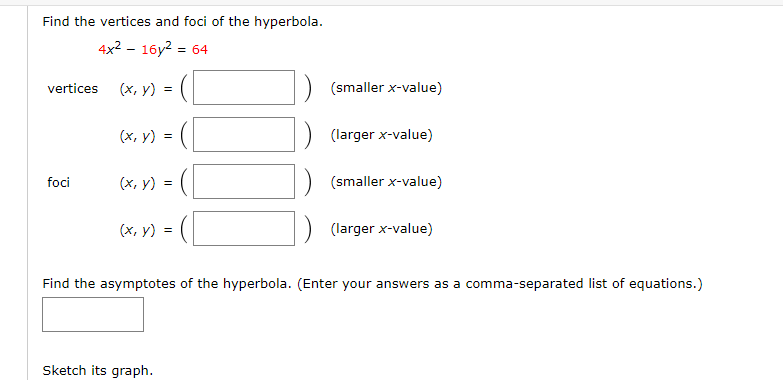# Find the vertices and foci of the hyperbola. 4x2 - 16y2 = 64 vertices (х, у) (smaller x-value) (х, у) (larger x-value) (x, y) = ( (smaller x-value) foci (х, у) %3D (larger x-value) Find the asymptotes of the hyperbola. (Enter your answers as a comma-separated list of equations.) Sketch its graph.

Question

10.5help_outlineImage TranscriptioncloseFind the vertices and foci of the hyperbola. 4x2 - 16y2 = 64 vertices (х, у) (smaller x-value) (х, у) (larger x-value) (x, y) = ( (smaller x-value) foci (х, у) %3D (larger x-value) Find the asymptotes of the hyperbola. (Enter your answers as a comma-separated list of equations.) Sketch its graph. fullscreen